SEARCH HOMEMath Central Quandaries & QueriesQuestion from Claire, a parent: Given one side of a triangle is 4 cm and the ratio 1:3 for the other 2 sides. What is the largest area of the triangle?Hi Claire.

This formula will be our starting point for your question:

 Heron's Formula for the area of any triangle: Given the lengths a, b and c of the sides of a triangle, the area A of the triangle is: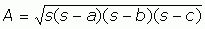where the value s is the semi-perimeter of the triangle. In other words,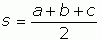.

For your triangle, you have sides of length 4, x and 3x, so: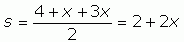and therefore: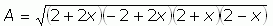.

Now if we multiply this out, we get: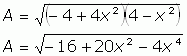This looks awful, but since the largest value of A will also lead to the largest value of A2, why don't we just square both sides? I'll also factor out a -4.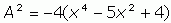If you look carefully at what is inside the parentheses, you can see that this is a quadratic in x2. What that means is that we can think of y = x2, so we have y2 - 5y + 4. That's just a normal quadratic. So we can "complete the square" next.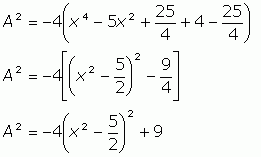Now look carefully at this equation. Because of the factor of -4, whatever is inside the parentheses is going to reduce A2. Since we want the maximum value of A2, we want the value inside the parentheses to be zero. You can easily work out the value of x that makes this so, but that isn't actually necessary in order to answer the question you've been asked. Simply, we know that inside the parentheses, the value can be zero, so we can ignore the first term entirely. Thus,

A2 = 9, so A = 3.

The maximum area is 3 square units.

Hope this helps you,
Stephen La Rocque and Harley Weston.Math Central is supported by the University of Regina and The Pacific Institute for the Mathematical Sciences.